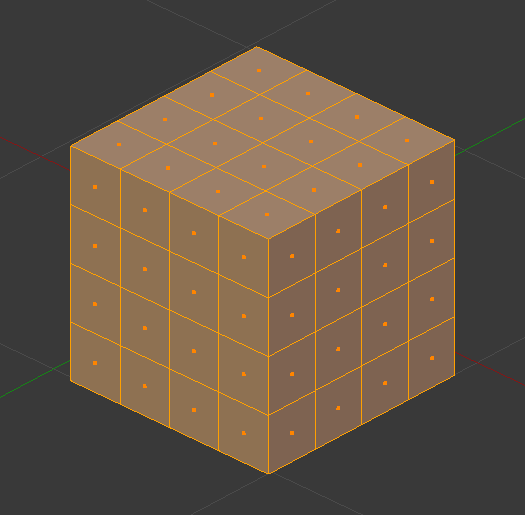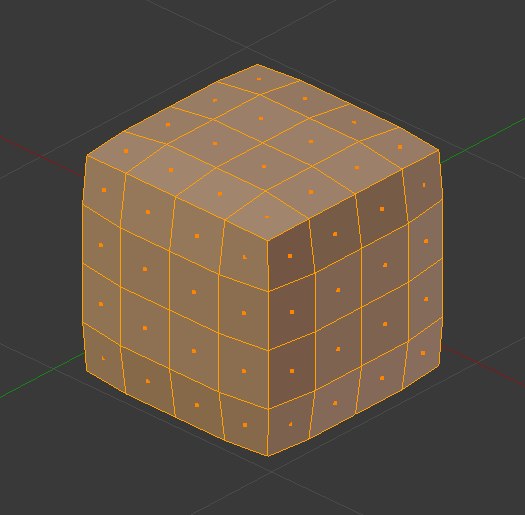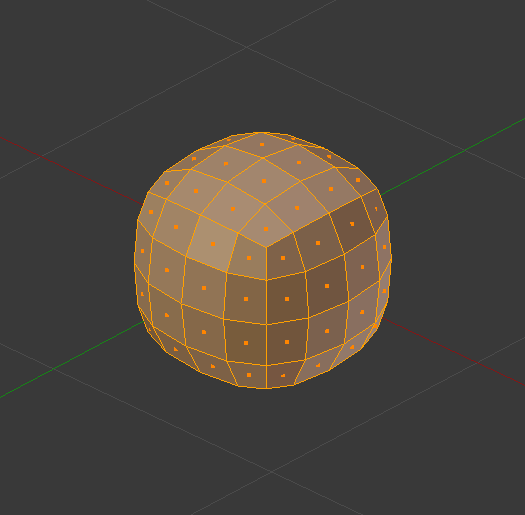The Smooth modifier smooths a mesh by flattening the angles between adjacent faces in it, just like the Smooth tool in Edit Mode. It smooths without subdividing the mesh, the number of vertices remains the same.

Esse modificador não é limitado a suavização, entretanto. Seu controle fator pode ser configurado fora da faixa (0.0 a 1.0) (incluindo valores negativos), que pode resultar em deformações interessantes.

## Opções

Eixos

Enable/disable the modifier in the X, Y and/or Z axes directions.

Fator

Controls the smoothing amount. Higher values will increase the effect.

Values outside expected range (above 1.0 or below 0.0) will distort the mesh.

Repetir

The number of smoothing iterations, equivalent to executing the Smooth tool multiple times.

Vertex Group

If set, restrict the effect to the only vertices in that vertex group. This allows for selective, real-time smoothing, by painting vertex weights.

Inverter `<->`

Inverte a influência do grupo de vértices selecionado, o que significa que o grupo agora representa vértices que não serão deformados pelo modificador.

The setting reverses the weight values of the group.

## Algoritmo

The calculation done by the Smooth modifier is a simple and logical one, and can be thought of as the geometric equivalent of blurring images.

Each new vertex position is simply moved towards the average position of all its neighbor vertices (topologically speaking, i.e. the vertices directly connected to it by an edge).

## ExemploMalha antes da suavização.Malha depois de uma iteração de suavização.Malha após dez iterações de suavização.